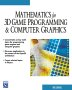# Maths - Matrix Diagonalisation

Some matrices can be transformed to diagonal matrices, that is, a matrix where the terms not on the leading diagonal are zero.

For a symmetrical matrix we can rotate it to get a diagonal matrix, do some operation, then rotate it back to its original coordinates. This rotation matrix is the eigen matrix or the orthonormal basis of [A], in other words:

[D] = [Q]-1 [A] [Q]

where:

• [D] = Diagonal matrix, diagonal terms are eigenvectors of A
• [A] = Symmetrical Matrix
• [Q] = Orthogonal matrix, columns are eigenvectors of A
• [Q]-1 = inverse of [Q]

## Derivation

The length of a vector squared is given by:

|V|² = Vt * V

where

• V = vector
• Vt = transpose of vector
• |V| = length of vector

This length will be unchanged if the coordinates are rotated by a matrix [R]. In this case the vector V is replaced by [R]V and the transposed vector Vt is replaced by Vt[R]t (transposing both operands reverses the order) and the unchanged length is therefore:

|V|² = Vt * V = Vt[R]t[R]V

therefore:

[R]t[R] = 

where

•  = identity matrix
• [R]t = transpose of rotation matrix

## Inertia Tensor

An example of diagonalisation is an inertia tensor.

1. Find the eigenvalues a by solving 0 = det{[A] - a) for a. The values of a are the principal moments of inertia.
2. Find the eigenvectors v of A by solving A v = a v for v.
3. Normalize the eigenvectors.
4. Form the matrix C whose whose columns consist of the normalized
5. D = Ct A C is the diagonal matrix of principal moments of inertia.

In principle, you can write down D directly after (1), however, completing (1) to (5) gives a check on your work.

Note: Ct is the transpose of C.

For this case where the only off diagonal terms are 12 and 21, you
know it only needs a rotation about axis 3 to diagonalize it. Use a
similarity transformation:
A'JA where A is the 3x3 rotation matrix about z.
Solve to find
j12 = 0 = j11cos²(a) -j22sin²(a) solve for a
j22 = j22cos²(a) - j11sin²(a)
j11 = j11cos²2(a) - j22sin²(a)
I don't know if that's easier.

 metadata block see also: Correspondence about this page Book Shop - Further reading. Where I can, I have put links to Amazon for books that are relevant to the subject, click on the appropriate country flag to get more details of the book or to buy it from them.Mathematics for 3D game Programming - Includes introduction to Vectors, Matrices, Transforms and Trigonometry. (But no euler angles or quaternions). Also includes ray tracing and some linear & rotational physics also collision detection (but not collision response). Other Math Books Specific to this page here:

This site may have errors. Don't use for critical systems.